# 10. PTM2, C3 – The Error Ratio

## 10.1 – Who is X and who is Y?

I hope the previous chapter gave you a basic understanding of linear regression and how one can conduct the linear regression operation on two sets of data, on MS Excel. Remember, we are talking about two variables here – X and Y.

X is defined as the independent variable and Y is the dependent variable. If you’ve spent time thinking about this, then I’m certain you’d have guessed X and Y will eventually be two different stocks.

In fact, let us just go ahead and run a linear regression on two stocks – maybe HDFC Bank and ICICI Bank and see what results we get.

I’m setting ICICI Bank as X and HDFC Bank as Y. A quick note on data before we proceed –

1. Make sure your data is clean – adjusted for splits, bonuses, and any other corporate actions
2. Make sure the data matches the exact dates – for instance, the data I have for both the stocks here runs from 4th of Dec 2015 to 4th Dec 2017.

Here is how the data looks –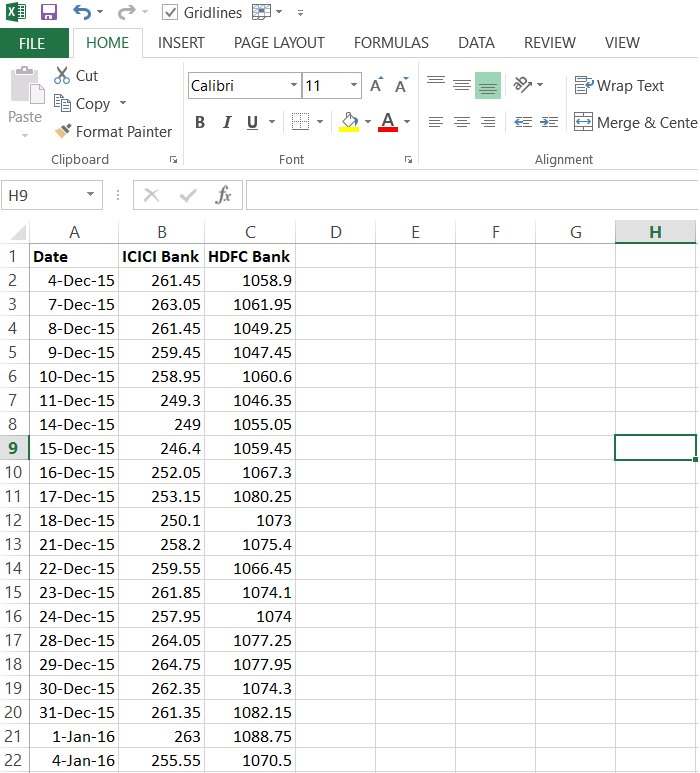I’ll run the linear regression on these two stocks (I’ve explained how to do this in the previous chapter), also do note, I’m running this on the stock prices and not really on stock returns –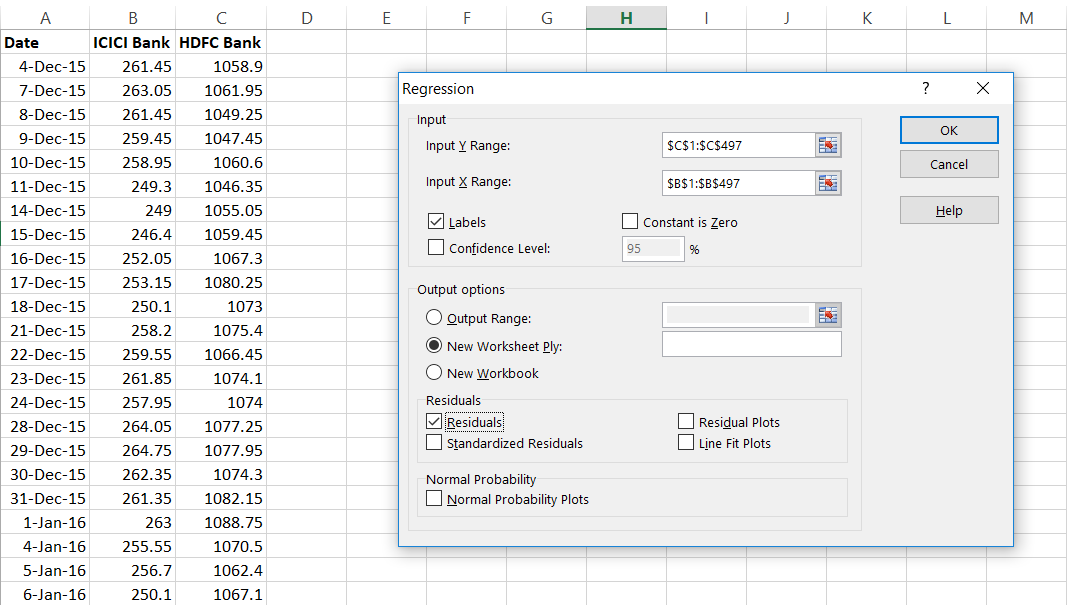The result of the linear regression is as follows –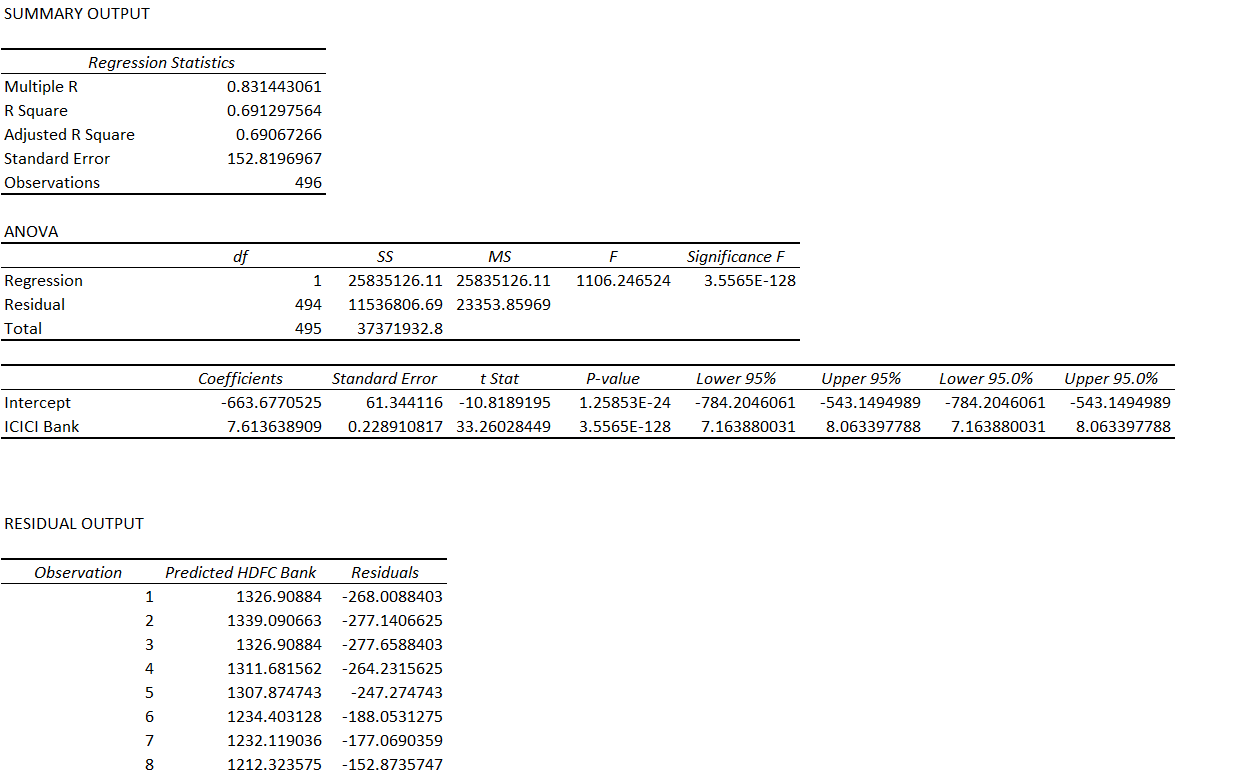Since ICICI is independent and HDFC is dependent, the equation is –

HDFC = Price of ICICI * 7.613 – 663.677

I’m assuming, you are familiar with the above equation.  For those who are not familiar, I’d suggest you to read the previous two chapters. However here is the quick summary – the equation is trying to predict the price of HDFC using the price of ICICI.

Or in other words, we are trying to ‘express’ the price of HDFC in terms of ICICI.

Now, let us reverse this – I will set ICICI as dependent and HDFC as the independent.

Here is how the results look –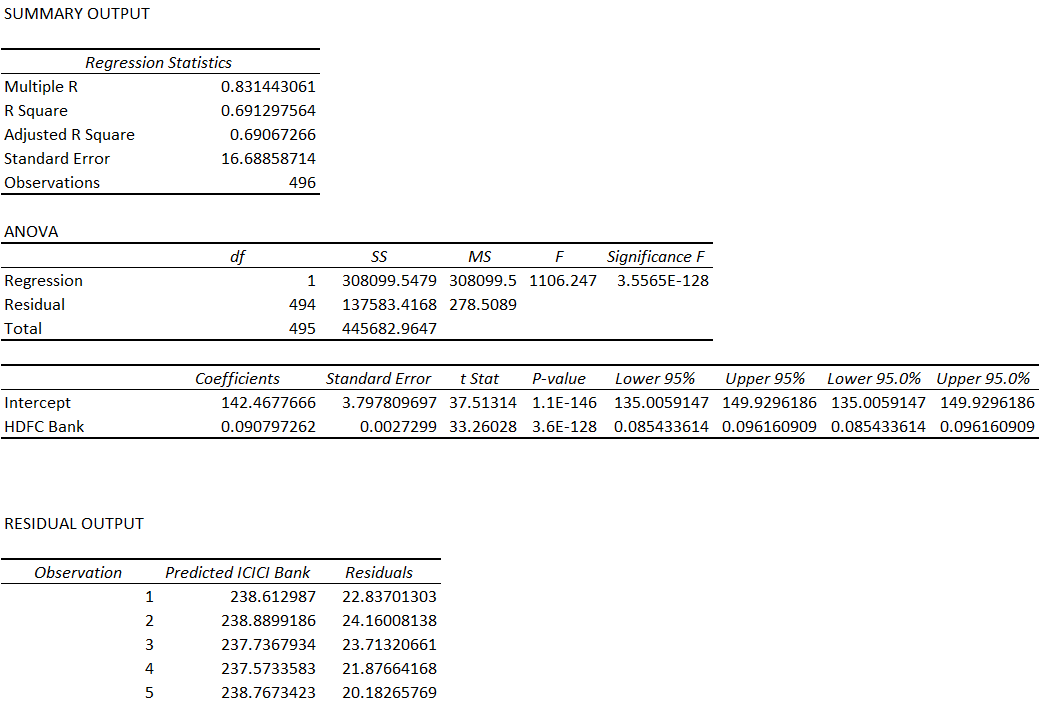The equation is –

ICICI = HDFC * 0.09 + 142.4677

So for the given two stocks, you can regress two ways by reordering which stock is dependent and which one is the independent variable.

However, the question is – how do you decide which one should be marked dependent and which one as independent. Or in other words, which order makes the most sense.

The answer to this depends on three things –

1. Standard Error
2. Standard Error of intercept
3. The ratio of the above two variables.

Remember, the linear equation above, essentially express the variation of price of ICICI in terms of HDFC (refer to the equation above). This expression or explanation of the price variation of one stock by keeping the price of the other stock as a reference can never be 100%. If it was 100%, then there is no play here at all.

Having said so, the equation should be strong enough to explain the variation in price of the dependent variable as much as possible, keeping the independent variable in perspective. The stronger this is, the better it is.

This leads us to the next obvious question – how do we figure out how strong the linear regression equation is? This is where the ratio –

Standard Error of Intercept / Standard Error comes into play.  To understand this ratio, we need to understand both the numerator and the denominator before talking about the ratio itself.

## 10.2 – Back to residuals

Here is the linear regression equation of ICICI as independent and HDFC as the dependent –

HDFC = Price of ICICI * 7.613 – 663.677

This essentially means, if I know the price of ICICI, I should be able to predict the price of HDFC. However, in reality, there is a difference between the predicted price of HDFC and the actual price. This difference is called the ‘Residuals’.

Here is the snapshot of the residuals when we try and explain the price of HDFC keeping ICICI as the independent variable –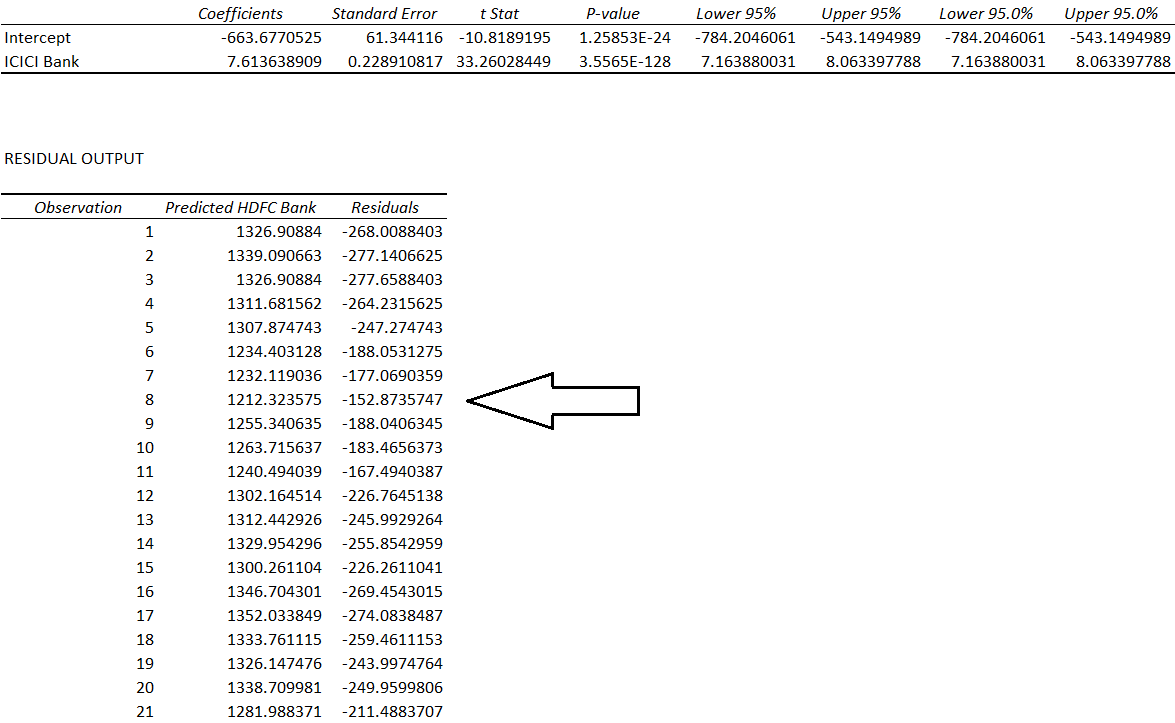When I talk about the regression equation and the residuals, usually, I get one common question – what is the use of regression if there is a residual each and every time? Or in other words, how can we rely on an equation, which fails to predict accurately, even once.

This is a fair question. If you look at the residuals above, they vary from a low of -288 to a high of 548, so using this equation to make any sort of prediction one price is futile.

But then, this was never about predicting the price of the dependent stock, given the price of an independent stock. It was always about the residuals!

Let me give you a heads-up here – the residuals display a certain behaviour.  If we can understand this behaviour and figure a pattern within it, then we can rework backwards to construct a trade. This trade obviously involves buying and selling the two stocks simultaneously, hence this qualifies as a pair trade.

Over the next few chapter, we will dwell deeper into this. However, for now, let’s talk about the ‘Standard Error’, the denominator in the Standard Error of Intercept / Standard Error equation.

The standard error is one of the variables which gets reported when you run a linear regression operation. Here is the snapshot showing the same –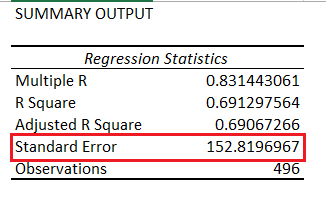The standard error is defined as the standard deviation of the residuals. Remember, the residuals itself is a time series array. So if you were to calculate the standard deviation of the residuals, then you get the standard error.

In fact, let me manually calculate the standard error of the residuals, I’m doing this for X = ICICI and y = HDFC

And excel tells me the standard deviation is 152.665. The standard error as reported in the summary output is 152.819. The minor difference can be ignored.

The ‘Standard Error of the Intercept’, is a little tricky. It does get reported in the regression report, and here is the standard error of the intercept with x = ICICI and y = HDFC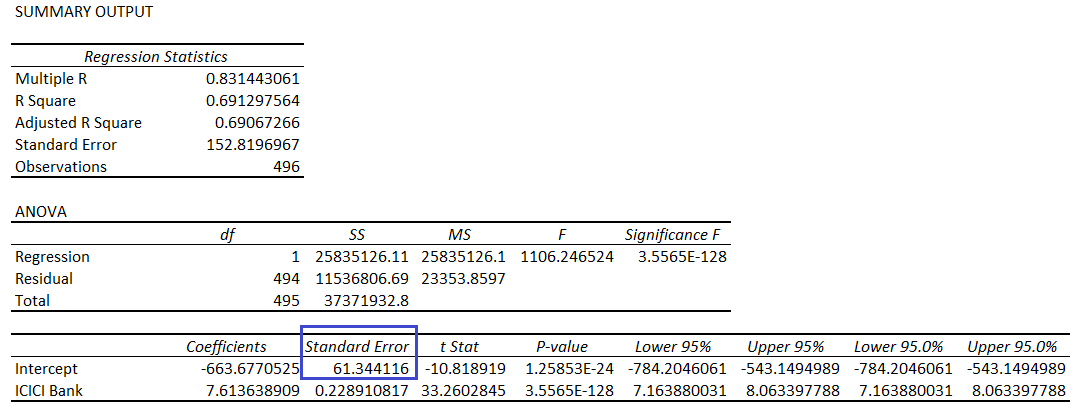Recall, the regression equation –

y=M*x+ C

Where,

M = Slope

C = Intercept

If you realize, here both M and C are estimates. And how are they estimated? They are estimated based on the historical data provided to the regression algorithm. The data can obviously contain noise components and few outliers. This implies that there is a scope for the estimates can go wrong.

The Standard Error of the Intercept is the measure of the variance of estimated intercept. It helps up understand by what degree the intercept itself can vary. So in a sense, this is somewhat similar to the ‘Standard Error’ itself. To summarize –

• Standard Error of Intercept – The variance of the intercept
• Standard Error – The variance of the residuals.

Now that we have defined both these variables, let’s bring back the ‘Error Ratio’. Please note, the term ‘Error Ratio’ is not a standard term, I’ve come up with it for ease of understanding.

Anyway, the error ratio, as we know –

Error Ratio = Standard Error of Intercept / Standard Error

I’m calculated the same for –

1. ICICI as X and HDFC as y = 0.401
2. HDFC as X and ICICI as y = 0.227

The decision to designate X and Y to stocks depends on the value of the error ratio. The lower the better. Since HDFC as X and ICICI as y offers the lowest error ratio, we will designate HDFC as the independent variable (X) and ICICI as the dependent variable (Y).

I’d love to explain the reason as to why we are using the error ratio as the key input for designating X and Y, but I guess I will hold back. I’ll revisit this again when I take up pair trade example.

For now, remember to calculate the error ratio and estimate which stock should be dependent and which one will be the independent.

## Key takeaways from the this chapter

1. X is the independent stock and Y is the dependent stock
2. The decision to figure out which stock is X and which one should be Y depends on ‘Error Ratio’
3. Both the slope and the intercept from the linear regression equation are estimates
4. Error Ratio = Standard Error of the Intercept / Standard Error
5. Standard error is the standard deviation of the residuals
6. Standard error of intercept gives you a sense of the variance of the intercept
7. Regress Stock 1 with Stock 2 and also Stock 2 with Stock 1, whichever offers the lowest error ratio defines which stock is dependent and which one is independent
8. Residuals display certain properties, studying which can help identify pair trading pattern

1.Deepu says:

Excellent write up Karthik!!!

The appetite to learn the complete thing on pair trade is at its peak. Please let us know when can we expect your next chapter. Eagerly waiting.

Thank a million once again.

Regards
Deepu

•Karthik Rangappa says:

Thanks for the kind comment, Deepu. I’ll try and put up the next chapter soon.

2.rohit jain says:

Kite 3 platform has the function to find correlation coefficient ratio between two stocks. For eg. correlation between Banknifty & Yesbank in Excel function Correl() returns a value of .38, Kite 3 shows the ratio as .83. Why the difference?

•rohit jain says:

Its calculated for last 1 year

•Karthik Rangappa says:

Yes, but you can calculate this for 2 years, 1 year, and 6 months.

•Karthik Rangappa says:

Rohit, can you tell me which study you are referring to in Kite3?

•rohit jain says:

In Kite 3, in “Chart”, under “Studies” menu, i selected “Correlation Coefficient” to find for stocks between banknifty and yesbank. In Kite 3, its showing all the same as .83 for 3 months, 6 months, 1 year. Strange. In excel, correl() functions, its .38 for 1 year and -.02 for 6 months. How the answers are coming different?

•Karthik Rangappa says:

Let me check this, Rohit.

3.Sundeep says:

Sir I think I’ve read this mean reverting strategy in a website called Quantopian but I could never understand it before now. Thank you. My question is, do you think its possible to create new mean reverting strategies if one has a firm understanding of statistical methods. You think a retail investor can do that?

•Karthik Rangappa says:

Yes, you certainly can. These are not latency critical strategies, meaning, the speed of information does not matter. What matters is the statistical/ quantitative technique. As long as you have a firm understanding of this – there are always opportunities in the market.

4.Akash Patel says:

Sir,
Can we keep the simple method based on correlation and cointegration only? This chapters going to much deeper now.
First i find 80% or more corelated stocks pair for last 63days (one quarter) .then check cointegration, in which pair cointegration less then 0.5 for last one year..i simple create the trade and its working fine. My favorite pairs are banknifty/nifty, acc/ambuja and tatamotors/tatamtrdvr…
I.cant understand why u taking us in too deep…

•Deepu says:

Akash

With all due respect to your views, please don’t mind but my view is that to have all the tools in place for any trading strategies.

Trust me knowledge is power and the deeper we are into learning and upskilling we will be in positive side of making money.

These all different statistics tools which will make the trades better or least we can avoid bad trades.

Simplicity is good but when we are dealing with Biggies than we need to be as smart as possible on the knowledge front.

Once again thanks Karthik for sharing the knowledge. Your sharing attitude is priceless and so many countless people must be thankful to you for sharing the pearls of trading.

Thanks once again.

•Karthik Rangappa says:

Agree with you, Deepu 🙂

•rohit jain says:

Keep going Karthick, without allowing anyone to influence what you have set to do.

•Karthik Rangappa says:

Thanks, Rohit! Will do 🙂

•Karthik Rangappa says:

Akash, trust me, the method we are discussing here is a unique way to pair trade. You will not find this explanation anywhere else. Learn it, and once you have all the information you can decide to adopt or ignore or even improvise the technique.

5.Akshay Hire says:

Sir, what if we use returns on two stocks rather than price to form pair trading strategies.

•Karthik Rangappa says:

You can, but that will lead to a very short-term approach to pair trade. This is best done with the price. Will try and give an explanation to this in the next chapter.